# Next Greater and Smaller Element for Every Element in an ArrayNeelakshi Lahiri
Last Updated: May 28, 2022
Difficulty Level :
MEDIUM

## Introduction

We all have learned about algorithms' time and space complexity and how it helps us write good code, but where do we use that knowledge practically?

Source: giphyTo solve problems, of course!

Coding rounds in the interviews of IT companies check a candidate’s ability to develop an efficient and less complex solution to problems. So to ace that round, we must be well versed with common questions and their best answers.

One common question is finding the next greater and next smaller element for every element in an array.

## Problem Statement

For a given array/list of integers of size N, print the next greater element(NGE) and the next smaller element(NSE) for every element.

• The next greater element for an element X is the first element on the right side of X in the array, which is greater than X.
• The next smaller element for an array element is the first element to the right of that element which has a value strictly smaller than that element.
• If no greater/smaller elements exist to the right of X, consider the next greater/smaller element as -1.

To understand what our question is, let us divide the question into two parts -

1. next greater and smaller element

2. for every element in an array

For example, consider an array,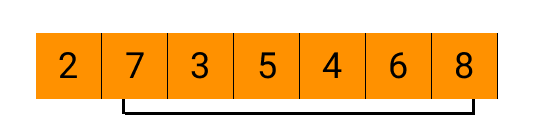Here the first element larger than 2 on its right side is 7, while there is no next smaller element after 2, so our output will be -1.

The second part of our questions says, “for every element in an array”, so we have to find the largest and smallest elements after each element.

Let us consider the same array shown above and find the next greater and smaller element for every element.

For 7,

Next greater element = 8 and next smaller element = 3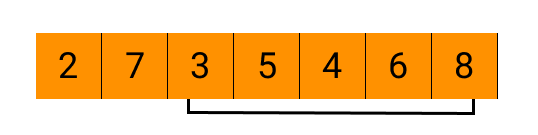For 3,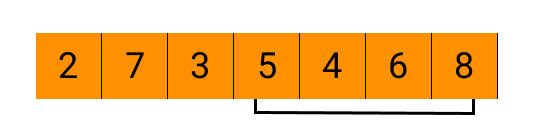Next greater element = 5 and next smaller element = -1

For 5,

Next greater element = 6 and next smaller element = 4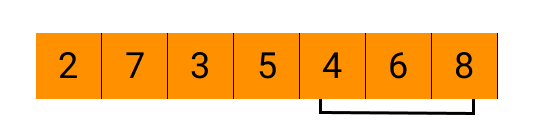For 4,

Next greater element = 6 and next smaller element = -1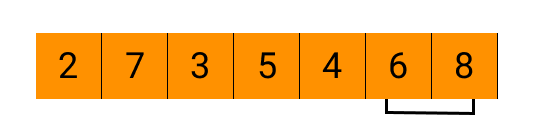For 6,

Next greater element = 8 and next smaller element = -1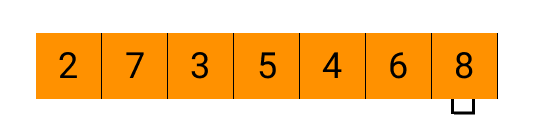For the last element, here 8, both the next greater and smaller elements will always be -1 since there is no element after it.

Thus, the final array with the next greater elements is:

Similarly, the final array with the next smaller elements is as follows: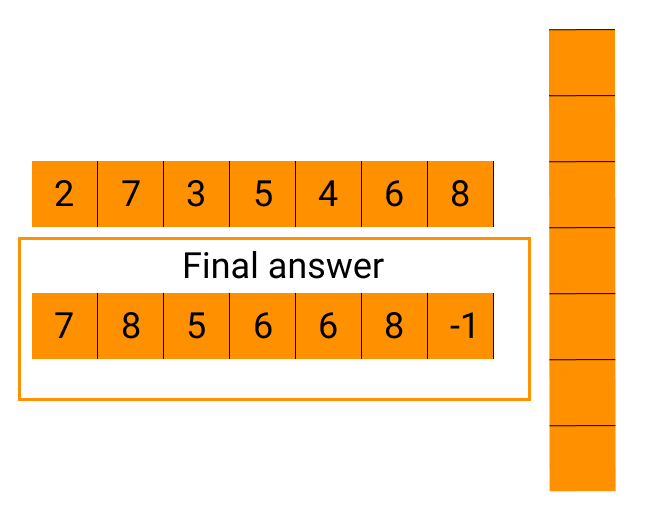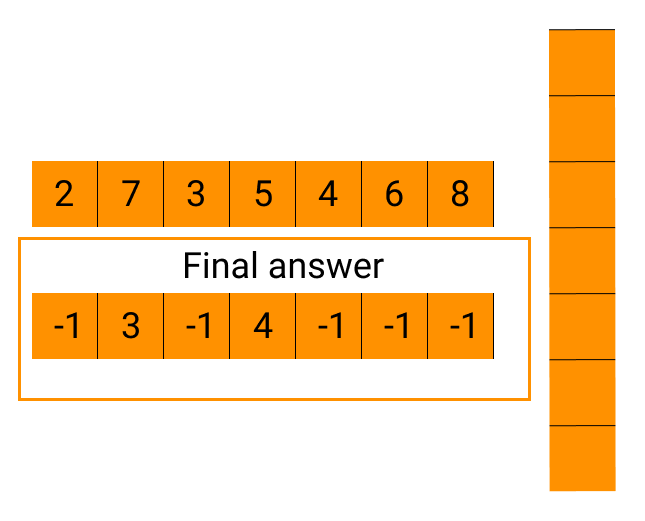Now that we’re clear with the problem we have to solve, let us see how we solve it.

## Method 1: Brute force

The simplest solution which comes to our mind is usually the brute force solution. Most of the time, this solution isn’t efficient with respect to the time and space complexity, so it isn’t preferred.

In the brute force solution to our problem, we will run a nested loop. The outer loop will traverse through each element in the array, while the inner loop will compare it with the elements after it to find the next greater and smaller element for every element.

At a glance, the method seems simple to think of and implement, but because of the nested loop, it has a time complexity of O(n2), which isn’t optimal.

Pretty easy yet complex, huh?

### Algorithm

Step 1: Start the outer loop from 0 to the array’s length and initialise a temporary variable (temp) with -1.

Step 2: Start the inner loop from i+1 to the size of the array.

Step 3: Check if the inner loop element is less than the outer loop element.

Step 4: If yes, print the element, assign 1 to temp and break out of the inner loop.

Step 5: Repeat the same procedure to find the next greater element for each element.

Step 6: Print the arrays “smaller” and “greater” as the answers.

### C++ Implementation

``````#include <iostream>
using namespace std;
void NextGreater(int arr[], int n){
for(int i=0;i<n;i++){
int temp=-1;
for(int j=i+1;j<n;j++){
if(arr[j]>arr[i]){
temp=arr[j];
cout<<temp<<" ";
break;
}
}
if(temp==-1){
cout<<temp<<" ";
}
}
}
void NextSmaller(int arr[], int n){
for(int i=0;i<n;i++){
int temp=-1;
for(int j=i+1;j<n;j++){
if(arr[j]<arr[i]){
temp=arr[j];
cout<<temp<<" ";
break;
}
}
if(temp==-1){
cout<<temp<<" ";
}
}
}
int main(){
int arr[]={2,13,10,6,3,9};
cout<<"Next Greater Element are"<<endl;
NextGreater(arr,6);
cout<<endl;
cout<<"Next Smaller Element are"<<endl;
NextSmaller(arr,6);
}``````

Output

``````Next Greater Element are
13 -1 -1 9 9 -1
Next Smaller Element are
-1 10 6 3 -1 -1``````

### Java Implementation

``````class Main
{
// Find the next greater element for every array element
public static void findNextGreater_And_SmallerElements(int[] input)
{
// base case
if (input == null) {
return;
}

// do for each element
for (int i = 0; i < input.length; i++)
{
// keep track of the next greater element for element `input[i]`
int next = -1;

// process elements on the right of element `input[i]`
for (int j = i + 1; j < input.length; j++)
{
// break the inner loop at the first larger element on the
// right of element `input[i]`
if (input[j] > input[i])
{
next = input[j];
break;
}
}

System.out.print(next + " ");
}
System.out.println();
for (int i = 0; i < input.length; i++)
{
// keep track of the next greater element for element `input[i]`
int next = -1;

// process elements on the right of element `input[i]`
for (int j = i + 1; j < input.length; j++)
{
// break the inner loop at the first larger element on the
// right of element `input[i]`
if (input[j] < input[i])
{
next = input[j];
break;
}
}

System.out.print(next + " ");
}

}

public static void main(String[] args)
{
int[] input = {2,13,10,6,3,9};
findNextGreater_And_SmallerElements(input);
}
}``````

Output

``````13 -1 -1 9 9 -1
-1 10 6 3 -1 -1``````

### Python Implementation

``````
def findNextGreaterElements(input):

# base case
if not input:
return

# do for each element
for i in range(len(input)):
# keep track of the next greater element for element `input[i]`
next = -1
# process elements on the right of element `input[i]`
for j in range(i + 1, len(input)):
# break the inner loop at the first larger element on the
# right of element `input[i]`
if input[j] > input[i]:
next = input[j]
break

print(next, end=' ')

if __name__ == '__main__':

input = [2,13,10,6,3,9]
findNextGreaterElements(input)``````

Output

``13 -1 -1 9 9 -1``

The Time Complexity- O(n*n)

This method isn’t the best due to its O(n2) time complexity. So now you may be wondering, what is the best approach? Let’s find out!

## Approach 2: Using Stack

In this method, we will have to traverse through the array only once because of the stack. Let us understand how that works by finding the next greater element.

Here, we will have a counter, say i, which will traverse through the array,

an output array to store answers (of the same length as the given array) initialized with -1 and an empty stack.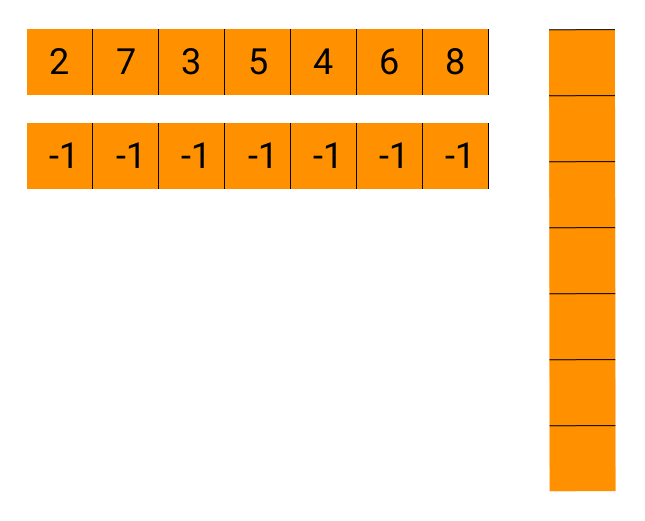To begin with, when the stack is empty, the first element of the array will be pushed into it.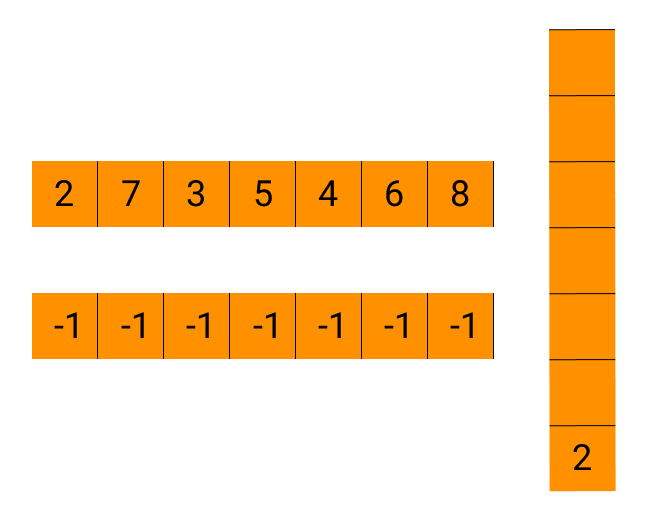Once there is an element in the stack, we will check whether the current element from the array is greater than the top element in the stack.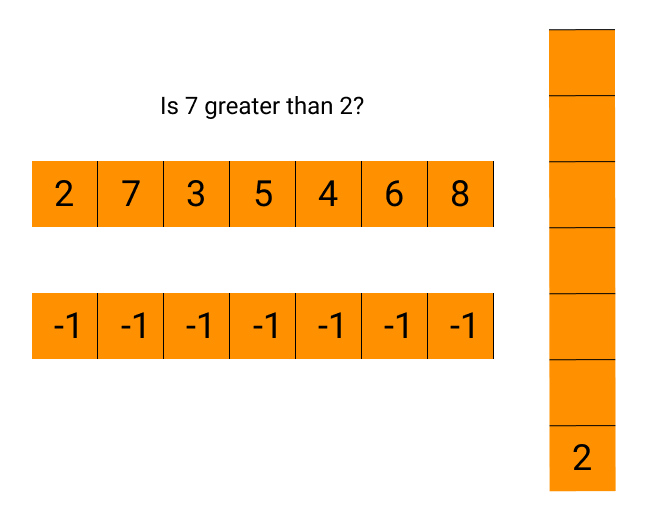If yes, pop the element from the stack, and store the current element from the array as the next greater element. Continue checking and popping until the top < current element or the stack is empty.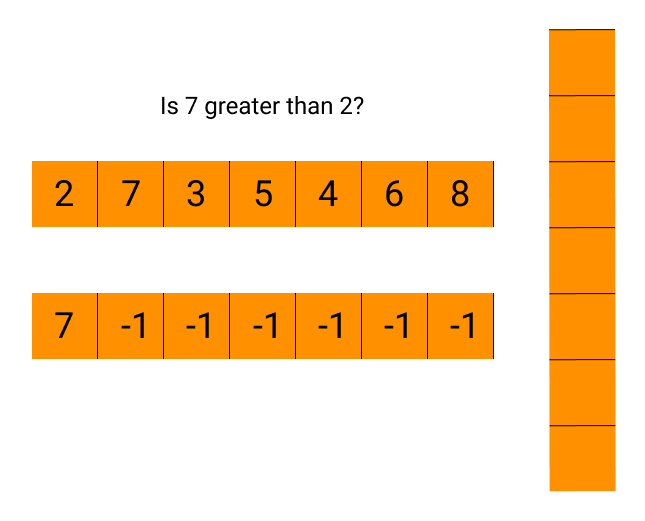After that, push the current element from the array into the stack.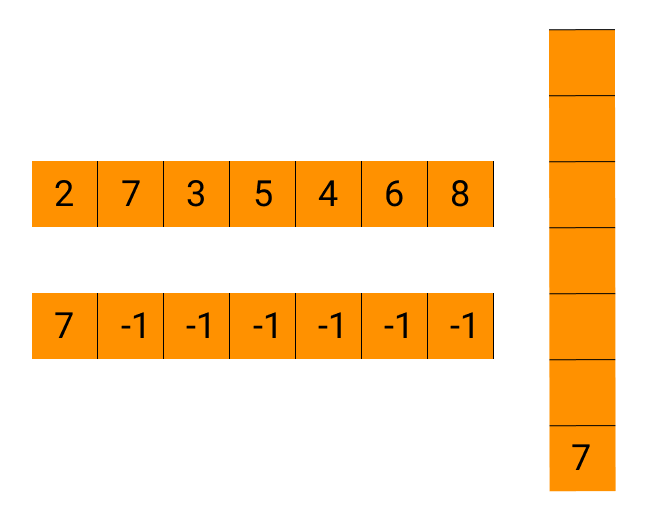For the next element in the array,Here, 3 isn’t greater than 7, so we will push it.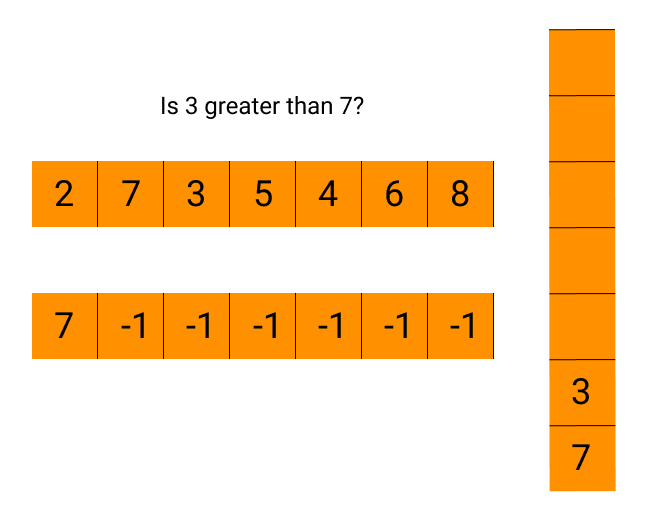For the subsequent elements,As we can see, the final answer matches what we had seen in the problem statement and the brute force solution.

We can also find the next smaller element similarly by just changing the checking condition.

### Algorithm

Step 1: Initialise an array, say answer (with the same size as the given array), with -1, and create a stack.

Step 2: In a loop, start traversing through the array.

Step 3: If the stack isn’t empty and the current element in the array is greater than the top element in the stack, pop the elements from the stack. Also, add the current element in the array to the answer array in the corresponding positions.

Step 4: After the condition mentioned above is not fulfilled, push the current element from the array into the stack.

Step 5: Return the answer array, which contains the next greater elements for each element.

Step 6: Repeat all the steps mentioned above to find the next smaller element for each element, replacing the “greater than” condition with “less than” in step 3.

### C++ Implementation

``````#include<bits/stdc++.h>

vector<int> findNextGreaterElements(vector<int>&arr)
{

int n=arr.size();

stack<int> s;   //creating an empty stack
for (int i = 0; i < n; i++)   //traversing through the elements in the array
{
while (!s.empty() && arr[s.top()] < arr[i])   //finds next greater element
{
}
s.push(i);
}
}

//Method to find the next smaller element for every array element
vector<int> findNextSmallerElements(vector<int>&arr)
{
int n=arr.size();

stack<int> s;   //creating an empty stack
for (int i = 0; i < n; i++)   //traversing through the elements in the array
{
while (!s.empty() && arr[s.top()] > arr[i])   //finds next smaller element
{
}
s.push(i);
}
}

int main()
{
int n,x;
cin>>n;
vector<int>input;
for(int i=0;i<n;i++){
cin>>x;
input.push_back(x);
}
vector<int>result = findNextGreaterElements(input);
cout<<"Next Greater Elements are"<<endl;
for(auto a:result){
cout<<a<<" ";
}
cout<<endl;
result = findNextSmallerElements(input);
cout<<"Next Greater Elements are"<<endl;
for(auto a:result){
cout<<a<<" ";
}
}``````

Output

``````Next Greater Elements are
7 8 5 6 6 8 -1
Next Smaller Elements are
-1 3 -1 4 -1 -1 -1``````

### Java Implementation

``````
import java.util.Arrays;
import java.util.Stack;
class Main
{
//Method to find the next greater element for every array element
public static int[] findNextGreaterElements(int[] arr)
{
if (arr == null)   //checks if the array is empty
{
return arr;
}
Stack<Integer> s = new Stack<>();   //creating an empty stack
for (int i = 0; i < arr.length; i++)   //traversing through the elements in the array
{
while (!s.isEmpty() && arr[s.peek()] < arr[i])   //finds next greater element
{
}
s.push(i);
}
}

//Method to find the next smaller element for every array element
public static int[] findNextSmallerElements(int[] arr)
{
if (arr == null)   //checks if the array is empty
{
return arr;
}
int[] answer = new int[arr.length];   //creates the final array
Stack<Integer> s = new Stack<>();   //creating an empty stack
for (int i = 0; i < arr.length; i++)   //traversing through the elements in the array
{
while (!s.isEmpty() && arr[s.peek()] > arr[i])   //finds next greater element
{
}
s.push(i);
}
}

public static void main(String[] args)
{
int[] input = { 2, 7, 3, 5, 4, 6, 8 };
int[] result = findNextGreaterElements(input);
System.out.println("Next Greater Elements are");
System.out.println(Arrays.toString(result));
result = findNextSmallerElements(input);
System.out.println("Next Smaller Elements are");
System.out.println(Arrays.toString(result));
}
}``````

Output

``````Next Greater Elements are
7 8 5 6 6 8 -1
Next Smaller Elements are
-1 3 -1 4 -1 -1 -1``````

### Python Implementation

``````# Find the next greater element for every array element
def findNextGreaterElements(input):
# base case
if not input:
return

print("Next Greater Elements are")
# do for each element
for i in range(len(input)):
# keep track of the next greater element for element `input[i]`
next = -1
# process elements on the right of element `input[i]`
for j in range(i + 1, len(input)):
# break the inner loop at the first larger element on the
# right of element `input[i]`
if input[j] > input[i]:
next = input[j]
break
print(next, end=' ')

def findNextSmallerElements(input):
# base case
if not input:
return

print("Next Smaller Elements are")
# do for each element
for i in range(len(input)):
# keep track of the next greater element for element `input[i]`
next = -1
# process elements on the right of element `input[i]`
for j in range(i + 1, len(input)):
# break the inner loop at the first larger element on the
# right of element `input[i]`
if input[j] < input[i]:
next = input[j]
break
print(next, end=' ')

if __name__ == '__main__':
input = [2, 7, 3, 5, 4, 6, 8]
findNextGreaterElements(input)
findNextSmallerElements(input)
``````

Output

``````Next Greater Elements are
7 8 5 6 6 8 -1
Next Smaller Elements are
-1 3 -1 4 -1 -1 -1``````

### Time and space Complexity for all three programs

Time Complexity

O(N) as we are traversing the input array only once.

Space Complexity

O(N), as an extra stack is used to keep track of the elements.

### How do we find the next greater and smaller element for every element in an array?

There are two methods to find the next smaller and greater element for every element in an array - The brute force method and Using stack.

### What is the time complexity for the two methods to find the next greater and smaller element for every element?

The time complexity for the brute force method is O(n2), and the time complexity for the algorithm using stack is O(n).

### Which method is better to find the next greater and smaller element for every element?

The stack method is better to find the next greater and smaller element for every element in arrays.

### Why is the method using stack better to find the next greater and smaller element for every element?

The stack method is better since it has a linear time complexity (O(n)).

### What is the best complexity to find the next greater and smaller element for every element in an array?

The best complexity is O(n).

## Conclusion

This article taught us how to find the next greater and smaller element for every element in an array. As mentioned before, this is a question that is commonly asked in the coding rounds of interviews. So, our aim should be to have a complete understanding of the topic.

To further our knowledge, we should try writing the code ourselves. We can do that for the next greater element and the next smaller element separately on CodeStudio. Apart from this question, we will find many other practice problems common in coding interviews and the interview experience of people presently working in renowned product-based companies.

Happy learning!